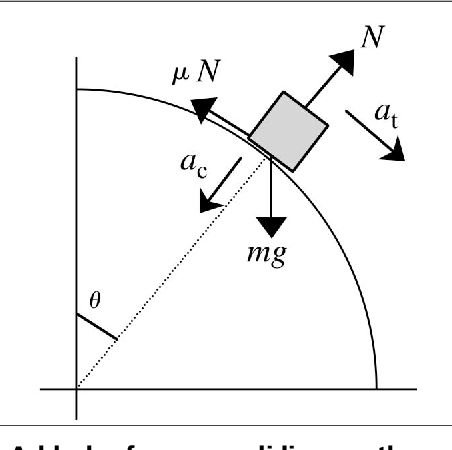## 耶鲁基础物理 7.2 万有引力- 阅读剩余部分 -

## 耶鲁基础物理 7.1 开普勒定律- 阅读剩余部分 -

## 耶鲁基础物理 6.6 重力势能- 阅读剩余部分 -

## 质点沿粗糙球面滑下的命运（补充内容后重发）- 阅读剩余部分 -

## 耶鲁基础物理 6.5 保守力- 阅读剩余部分 -

## 耶鲁基础物理5.4摩擦与动能定理- 阅读剩余部分 -

## 质点沿粗糙球面滑下的命运- 阅读剩余部分 -

## Mathematica 画受力示意图

 1 2 3 4 5 6 7 8 9 10 11 12 13 14 15 16 17 18
g1 = Graphics[Line[{{0, 0}, {20, 0}}]];g2 = Graphics[Line[{{0, 0}, {15, 15}}]];g3 = Graphics[{Opacity[0.2], Blue,     Rotate[Rectangle[{8, 8}, {12, 12}], 45 Degree, {Left, Bottom}]}];g4 = Graphics[{Blue, Thickness[0.01], Arrow[{{8, 10.8}, {8, 2.8}}]}];g5 = Graphics[{Cyan, Thickness[0.01], Arrow[{{8, 10.8}, {3.8, 15}}]}];g6 = Graphics[{Orange, Thickness[0.01],     Arrow[{{2.4, 5.2}, {6.6, 9.4}}]}];g7 = Graphics[   Text[StyleForm["重力", FontSize -> 14, FontWeight -> "Bold"], {9,      6.8}, {0, 1}, {0, -1}]];g8 = Graphics[   Text[StyleForm["支持力", FontSize -> 14, FontWeight -> "Bold"], {6,      13}, {0, -1}, {1, -1}]];g9 = Graphics[   Text[StyleForm["摩擦力", FontSize -> 14, FontWeight -> "Bold"], {4.5,      7.2}, {0, 1}, {1, 1}]];Show[g1, g2, g3, g4, g5, g6, g7, g8, g9]## 质点沿粗糙球面滑下的命运- 阅读剩余部分 -

## 标量、矢量和笛卡尔张量的解析定义\begin{equation} x'_i=\beta_{ij}x_j \label{x'x} \end{equation}

\begin{equation} x_i=\beta_{ji}x'_j \label{xx'} \end{equation}

\begin{equation} \Phi(x_1,x_2,x_3)=\Phi'(x'_1,x'_2,x'_3) \label{scalar} \end{equation}

\begin{equation} \begin{cases} \xi'_i(x'_1,x'_2,x'_3)=&\xi_i(x_1,x_2,x_3)\beta_{ik} \\ \xi_i(x'_1,x'_2,x'_3)=&\xi'_i(x_1,x_2,x_3)\beta_{ki} \end{cases} \label{vector} \end{equation}

\begin{equation} \begin{cases} t'_{ij}(x'_1,x'_2,x'_3)=&t_{mn}(x_1,x_2,x_3)\beta_{im}\beta_{jn} \\ t_{ij}(x_1,x_2,x_3)=&t'_{mn}(x'_1,x'_2,x'_3)\beta_{mi}\beta_{nj} \end{cases} \label{tensor} \end{equation}